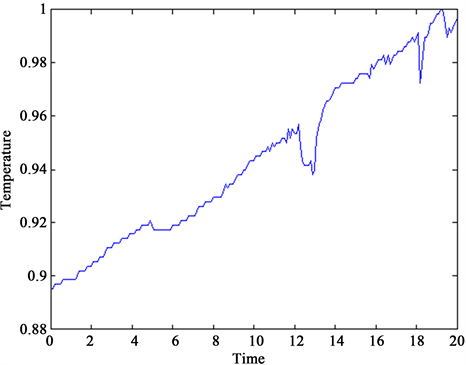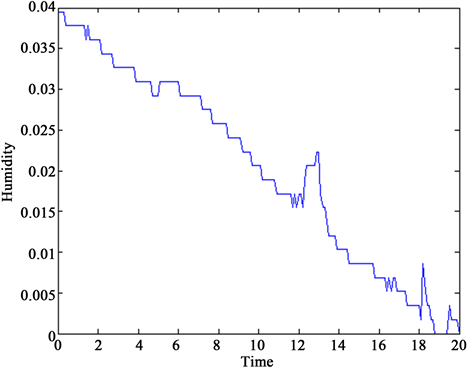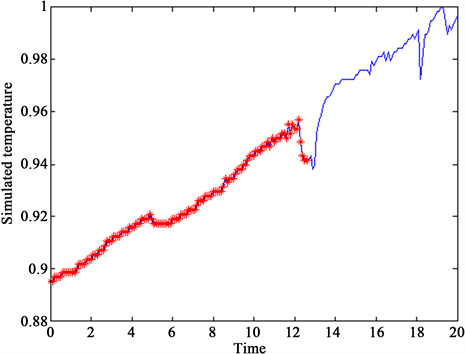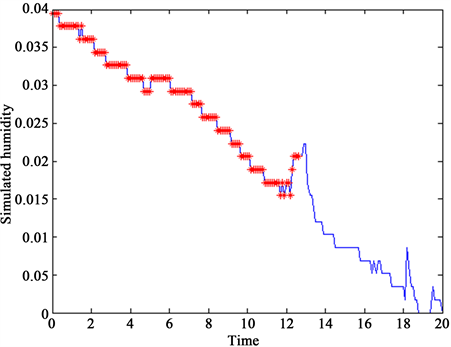﻿ 木材含水率的神经网络模型构建

# 木材含水率的神经网络模型构建Neural Network Model for Wood Moisture Content

Abstract: Wood moisture content is an important technical parameter in the wood drying process. In order to deal with data of the wood drying test more accurately and effectively, we decided to use the advantages of RMA neural network model to design an algorithm for wood moisture content in-fluencing factors and relationship of time. Firstly, we select a kind of neural network named Re-current Neural Network to set up a model algorithm. And next thing is building an operation pro-gram which can express the RMA neural network model well. Finally, we combine real empirical data with the program, and we get functional statements of systemic wood moisture content.

1. 引言

2. 实时建模算法RMA的理论建立

2.1. 实时建模算法RMA的基本思想

2.1.1. 非线性连续系统离散化

$\frac{\partial x\left(t,k\right)}{\partial t}=-\alpha x\left(t,k\right)+f\left({W}_{1}\left(t,k\right),x\left(t,k\right)\right)$ (1-1)

${W}_{1}\left(t,k+1\right)={W}_{1}\left(t,k\right)+\Delta {W}_{1}\left(t,k\right)$ (1-2)

2.1.2. 连接权矩阵的训练法

$e\left(t,k\right)=y\left(t\right)-x\left(t,k\right),t\in \left[0,\infty \right)$ (1-3)

$x\left(0,k\right)=x\left(0\right)=y\left(0\right),k=0,1,2,\cdots$ (1-4)

$\eta \left(t,k\right)={\int }_{0}^{t}\left[x\left(\tau ,k+1\right)-x\left(\tau ,k\right)\right]\text{d}\tau$ (1-5)

$\eta \left(0,k\right)=0$ ，由此可得

$\begin{array}{c}\frac{\partial \eta \left(t,k\right)}{\partial t}=x\left(t,k+1\right)-x\left(t,k\right)\\ =x\left(t,k+1\right)-x\left(0,k+1\right)-x\left(t,k\right)+x\left(0,k\right)\\ ={\int }_{0}^{t}\frac{\partial x\left(\tau ,k+1\right)}{\partial \tau }\text{d}\tau -{\int }_{0}^{t}\frac{\partial x\left(\tau ,k\right)}{\partial \tau }\text{d}\tau \\ =-\alpha {\int }_{0}^{t}x\left(\tau ,k+1\right)\text{d}\tau +{\int }_{0}^{t}f\left({W}_{1}\left(\tau ,k+1\right),x\left(\tau ,k+1\right)\right)\text{d}\tau \\ \text{\hspace{0.17em}}\text{\hspace{0.17em}}\text{ }+\alpha {\int }_{\text{0}}^{t}x\left(\tau ,k\right)\text{d}\tau -{\int }_{0}^{t}f\left({W}_{1}\left(\tau ,k\right),x\left(\tau ,k\right)\right)\text{d}\tau \\ =-\alpha \eta \left(t,k\right)+{\int }_{0}^{t}\left[f\left({W}_{1}\left(\tau ,k+1\right),x\left(\tau ,k+1\right)\right)-f\left({W}_{1}\left(\tau ,k\right),x\left(\tau ,k\right)\right)\right]\text{d}\tau \end{array}$

$\begin{array}{l}e\left(t,k+1\right)-e\left(t,k\right)\\ =x\left(t,k\right)-x\left(t,k+1\right)\\ =\alpha \eta \left(t,k\right)-{\int }_{0}^{t}\left[f\left({W}_{1}\left(\tau ,k+1\right),x\left(\tau ,k+1\right)\right)-f\left({W}_{1}\left(\tau ,k\right),x\left(\tau ,k\right)\right)\right]\text{d}\tau \end{array}$ (1-6)

$\begin{array}{l}f\left({W}_{1}\left(t,k+1\right),x\left(t,k+1\right)\right)-f\left({W}_{1}\left(t,k\right),x\left(t,k\right)\right)\\ ={K}_{1}\frac{\partial \eta \left(t,k\right)}{\partial t}+{K}_{2}\frac{\partial e\left(t,k\right)}{\partial t}\end{array}$ (1-7)

$\left[\begin{array}{c}\frac{\partial \eta \left(t,k\right)}{\partial t}\\ e\left(t,k+1\right)\end{array}\right]=\left[\begin{array}{cc}-\alpha I+{K}_{1}& {K}_{2}\\ \alpha I-{K}_{1}& I-{K}_{2}\end{array}\right]\left[\begin{array}{c}\eta \left(t,k\right)\\ e\left(t,k\right)\end{array}\right]+\left[\begin{array}{cc}-{K}_{1}& -{K}_{2}\\ {K}_{1}& {K}_{2}\end{array}\right]\left[\begin{array}{c}\eta \left(0,k\right)\\ e\left(0,k\right)\end{array}\right]$ (1-8)

$\left[\begin{array}{c}\frac{\partial \eta \left(t,k\right)}{\partial t}\\ e\left(t,k+1\right)\end{array}\right]=\left[\begin{array}{cc}-\alpha I+{K}_{1}& {K}_{2}\\ \alpha I-{K}_{1}& I-{K}_{2}\end{array}\right]\left[\begin{array}{c}\eta \left(t,k\right)\\ e\left(t,k\right)\end{array}\right]$ (1-9)

$\left[\begin{array}{c}\frac{\partial x\left(t,k\right)}{\partial t}\\ y\left(t,k+1\right)\end{array}\right]=\left[\begin{array}{cc}{A}_{1}& {A}_{2}\\ {A}_{3}& {A}_{4}\end{array}\right]\left[\begin{array}{c}x\left(t,k\right)\\ y\left(t,k\right)\end{array}\right]$

$\underset{k\to \infty }{\mathrm{lim}}\left[\begin{array}{c}x\left(t,k\right)\\ y\left(t,k\right)\end{array}\right]=0,t\in \left[0,T\right]$

2.2. 非线性连续系统的建模算法

$f\left({W}_{1}\left(t\right),x\left(t\right)\right)={W}_{1}\sigma \left(x\left(t\right)\right)，f\left({W}_{1}\left(t\right),x\left(t\right)\right)=\sigma \left({W}_{1}x\left( t \right) \right)$

$f\left({W}_{1}\left(t\right),x\left(t\right)\right)={W}_{1}\left(t\right)\sigma \left(x\left(t\right)\right)$ (1-10)

$\begin{array}{l}{W}_{1}\left(t,k\right)\sigma \left(x\left(t,k+1\right)\right)+\Delta {W}_{1}\left(t,k\right)\sigma \left(x\left(t,k+1\right)\right)-{W}_{1}\left(t,k\right)\sigma \left(x\left(t,k\right)\right)\\ ={K}_{1}\frac{\partial \eta \left(t,k\right)}{\partial t}+{K}_{2}\frac{\partial e\left(t,k\right)}{\partial t}\end{array}$ (1-11)

$\begin{array}{l}\Delta {W}_{1}\left(t,k\right)=\left(\frac{\partial e\left(t,k\right)}{\partial t}+{W}_{1}\left(t,k\right)\left(\sigma \left(x\left(t,k\right)\right)-\sigma \left(x\left(t,k+1\right)\right)\right)\right)\\ \text{\hspace{0.17em}}\text{\hspace{0.17em}}\text{\hspace{0.17em}}\text{\hspace{0.17em}}\text{\hspace{0.17em}}\text{\hspace{0.17em}}\text{\hspace{0.17em}}\text{\hspace{0.17em}}\text{\hspace{0.17em}}\text{\hspace{0.17em}}\text{\hspace{0.17em}}\text{\hspace{0.17em}}\text{ }\text{\hspace{0.17em}}\text{\hspace{0.17em}}\text{\hspace{0.17em}}\text{\hspace{0.17em}}\text{\hspace{0.17em}}×{\left({\left[\sigma \left(x\left(t,k+1\right)\right)\right]}^{\text{T}}\sigma \left(x\left(t,k+1\right)\right)\right)}^{-1}×{\left[\sigma \left(x\left(t,k+1\right)\right)\right]}^{\text{T}}\end{array}$ (1-12)

2.3. 实时建模算法RAM的实现

3. RMA建模算法处理数据及仿真程序实现

3.1. 利用MATLAB实现RMA建模算法

3.1.1. 程序构建思路

3.1.2. 数据形态

3.2. 木材含水率数据与RMA建模平台的融合Figure 1. Temperature and time at the experimental pointFigure 2. Humidity and time at the experimental pointFigure 3. Simulation of experimental data (temperature) by neural network modelFigure 4. Simulation of experimental data (humidity) by neural network model

4. 仿真实验结果及分析

$\left[\begin{array}{c}\text{d}{x}_{1}\\ \text{d}{x}_{2}\end{array}\right]=-A×\left[\begin{array}{c}{x}_{1}\\ {x}_{2}\end{array}\right]+\left[\begin{array}{cc}{w}_{11}& {w}_{22}\\ {w}_{33}& {w}_{44}\end{array}\right]×\left[\begin{array}{c}\mathrm{tanh}\left({t}_{1}\right)\\ \mathrm{tanh}\left({t}_{2}\right)\end{array}\right]$

$\begin{array}{l}{w}_{11}=-0.0008365{x}^{2}+0.0135x+0.4354\\ {w}_{22}=-0.0001135{x}^{2}+0.01734x+0.7403\\ {w}_{33}=0.0001015{x}^{2}-0.0003172x-0.01734\\ {w}_{44}=-0.0013{x}^{2}+0.0197x+0.8454\end{array}$

5. 结语

 张佳薇, 苏洪雨. 基于多模型建模的木材含水率测量方法研究[J]. 机电产品开发与创新, 2009, 22(5): 109-111.

 姜滨, 孙丽萍, 曹军, 等. GA优化T-S模糊神经网络的干燥窑温湿度控制器设计[J]. 实验室研究与探索, 2015, 34(11): 54-59.

 朱莉, 袁文爽, 尹文芳. 云遗传算法在木材干燥过程建模中的应用[J]. 机电产品开发与创新, 2009, 22(6): 99-101.

 王英, 曹军, 孙丽萍. 基于SAGA优化BP神经网络的木材含水率预测[J]. 自动化技术与应用, 2013, 32(1): 4-6.

 李晓东, 马玉韬. 用反馈神经网络对非线性离散系统进行实时建模[J]. 宁夏大学学报(自然科学版), 2000, 21(3): 229.

 杨国华, 李晓东. 利用反馈神经网络对非线性连续系统进行实时建模[J]. 宁夏大学学报(自然版), 2002, 23(3): 248-251.

 Kaczorek, T. (1994) Reachability and Con-trollability of 2-D Continuous Discrete Linear Systems. In: White, P.N., Ed., Symposium on Mathematical Models in Automation and Robotics, Miedzyzdroje, 24-28.

 Chow, T.W.S. and Fang, Y. (1998) An Iterative Learning Control Method for Continuous-Time Systems Based on 2-D System Theory. IEEE Transactions on Circuits & Systems I Fundamental Theory & Applications, 45, 683-689.
https://doi.org/10.1109/81.678496

Top